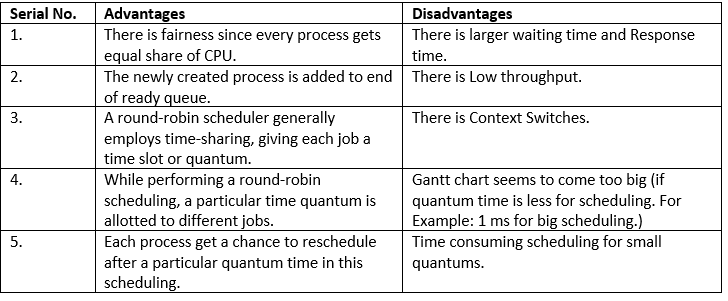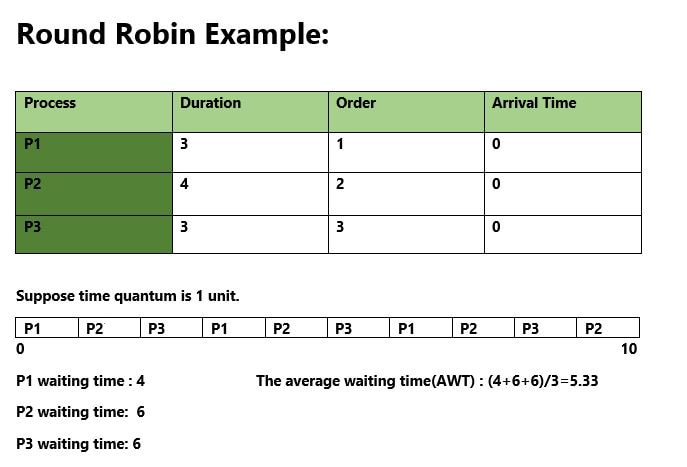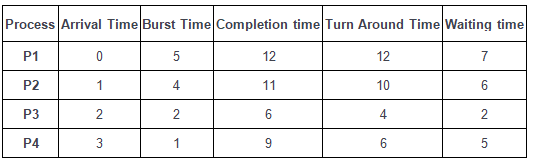Courses

# Round Robin Scheduling Notes | EduRev

## GATE : Round Robin Scheduling Notes | EduRev

The document Round Robin Scheduling Notes | EduRev is a part of the GATE Course Operating System.
All you need of GATE at this link: GATE

Program for Round Robin scheduling

Round Robin is a CPU scheduling algorithm where each process is assigned a fixed time slot in a cyclic way.

• It is simple, easy to implement, and starvation-free as all processes get fair share of CPU.
• One of the most commonly used technique in CPU scheduling as a core.
• It is preemptive as processes are assigned CPU only for a fixed slice of time at most.Illustration:How to compute below times in Round Robin using a program?

1. Completion Time: Time at which process completes its execution.
2. Turn Around Time: Time Difference between completion time and arrival time. Turn Around Time = Completion Time – Arrival Time
3. Waiting Time(W.T): Time Difference between turn around time and burst time.
Waiting Time = Turn Around Time – Burst Time

In this post, we have assumed arrival times as 0, so turn around and completion times are same.
The tricky part is to compute waiting times. Once waiting times are computed, turn around times can be quickly computed.
Steps to find waiting times of all processes:

1. Create an array rem_bt[] to keep track of remaining burst time of processes. This array is initially a copy of bt[] (burst times array)
2. Create another array wt[] to store waiting times of processes. Initialize this array as 0.
3. Initialize time : t = 0
4. Keep traversing the all processes while all processes are not done. Do following for i'th process if it is not done yet.
a- If rem_bt[i] > quantum
(i)  t = t + quantum
(ii) bt_rem[i] -= quantum;
c- Else // Last cycle for this process
(i)  t = t + bt_rem[i];
(ii) wt[i] = t - bt[i]
(iii) bt_rem[i] = 0; // This process is over

Once we have waiting times, we can compute turn around time tat[i] of a process as sum of waiting and burst times, i.e., wt[i] + bt[i]

Round Robin Scheduling with different arrival times

Round-robin scheduling algorithm is used to schedule process fairly each job a time slot or quantum and the interrupting the job if it is not completed by then the job come after the other job which is arrived in the quantum time that makes these scheduling fairly.

Note:

• Round-robin is cyclic in nature, so starvation doesn’t occur
• Round-robin is a variant of first come, first served scheduling
• No priority, special importance given to any process or task
• RR scheduling is also known as Time slicing scheduling

• Each process is served by CPU for a fixed time, so priority is the same for each one
• Starvation does not occur because of its cyclic nature.

• Throughput depends on quantum time.
• If we want to give some process priority, we cannot.Quantum time is 2 this means each process is only executing for 2 units of time at a time.

How to compute these process requests:

1. Take the process which occurs first and start executing the process(for quantum time only).
2. Check if any other process request has arrived. If a process request arrives during the quantum time in which another process is executing, then add the new process to the Ready queue
3. After the quantum time has passed, check for any processes in the Ready queue. If the ready queue is empty then continue the current process. If the queue not empty and the current process is not complete, then add the current process to the end of the ready queue.
4. Take the first process from the Ready queue and start executing it (same rules)
5. Repeat all steps above from 2-5
6. If the process is complete and the ready queue is empty then the task is complete

After all these we get the three times which are:

1. Completion Time: the time taken for a process to complete.
2. Turn Around Time: total time the process exists in the system. (completion time – arrival time).
3. Waiting Time: total time waiting for their complete execution. (turn around time – burst time ).

How to implement in a programming language

1. Declare arrival[], burst[], wait[], turn[] arrays and initialize them. Also declare a timer variable and initialize it to zero. To sustain the original burst array create another    array (temp_burst[]) and copy all the values of burst array in it.
2. To keep a check we create another array of bool type which keeps the record of whether a process is completed or not. we also need to maintain a queue array which contains the process indicies (initially the array is filled with 0).
3. Now we increment the timer variable until the first process arrives and when it does, we add the process index to the queue array
4. Now we execute the first process until the time quanta and during that time quanta, we check whether any other process has arrived or not and if it has then we add the index in the queue (by calling the fxn. queueUpdation()).
5. Now, after doing the above steps if a process has finished, we store its exit time and execute the next process in the queue array. Else, we move the currently executed process at the end of the queue (by calling another fxn. queueMaintainence()) when the time slice expires.
6. The above steps are then repeated until all the processes have been completely executed. If a scenario arises where there are some processes left but they have not arrived yet, then we shall wait and the CPU will remain idle during this interval.
Offer running on EduRev: Apply code STAYHOME200 to get INR 200 off on our premium plan EduRev Infinity!

69 docs|23 tests

,

,

,

,

,

,

,

,

,

,

,

,

,

,

,

,

,

,

,

,

,

;